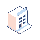• Pricing

# How do I calculate number of months between two dates ?

I have the need to find the number of months between two dates in python. Is there any standard python method to do that ?

1 CommentCan yo be more specific? How many months should be between april 30 and may 1st of the same year? And what about 1-st and last day of a specific month. And what about 15 of month x and 14 of month x+1?

You can try this:

``````from datetime import datetime
from dateutil import relativedelta
date1 = datetime.strptime(str('2011-08-15 12:00:00'), '%Y-%m-%d %H:%M:%S')
date2 = datetime.strptime(str('2012-02-15'), '%Y-%m-%d')
r = relativedelta.relativedelta(date2, date1)
r.months
``````

This will give you number of months between two dates.

Thanks, Priyesh Solankifor example:

``````from datetime import datetime
def days_between(d1, d2):
d1 = datetime.strptime(d1, "%Y-%m-%d")
d2 = datetime.strptime(d2, "%Y-%m-%d")
return abs((d2 - d1).days)

print days_between('2013-05-06', '2013-06-06')
``````Thank you for your answer, but I need to calculate number of month. The division by 30 doesn't give an exact result.

If you want the number of months you should use dateutil.relativedelta, as in Priyesh's answer. The reason for that is because it hides all the complex calculations required for different lengths of months, leap years, etc.

If I have inputs fields like date_from and date_to in pay slip calculation and i have to find the number of days between them . How can i use that ?? Can i use some variable of that kind in python code.?? Above code will work there??

I would like to refresh a bit this subject. Guys, how ... i tried Priyesh's answer but because I'm trying to provide date's from inputs ... it gives me an error: date1 = datetime.strptime(str('st_date'), '%Y-%m-%d %H:%M:%S') File "/usr/lib/python2.7/_strptime.py", line 325, in _strptime (data_string, format)) ValueError: time data 'st_date' does not match format '%Y-%m-%d %H:%M:%S' What to do with it ?

or this: date1 = datetime.strptime(str(st_date), '%Y-%m-%d %H:%M:%S') NameError: name 'st_date' is not defined 2015-12-21 21:48:00,534 3121 WARNING mydatabase openerp.sql_db: Cursor not closed explicitly Please enable sql debugging to trace the caller.